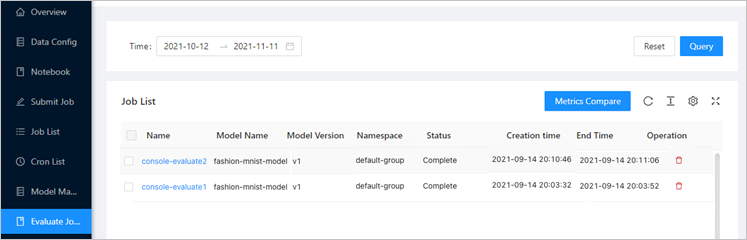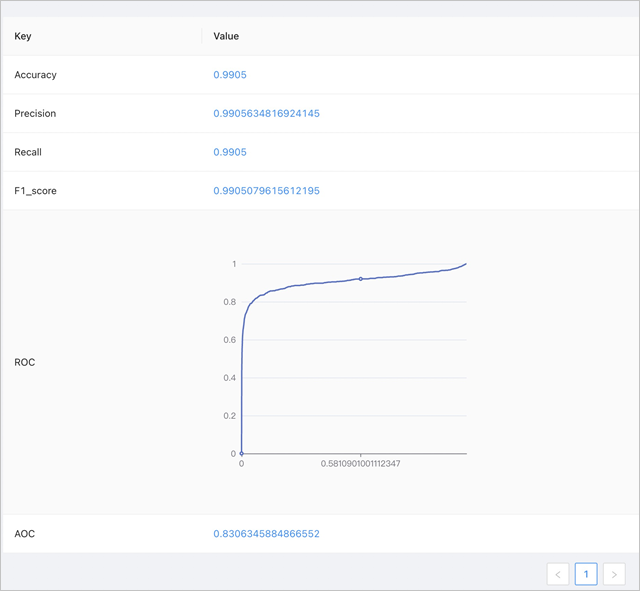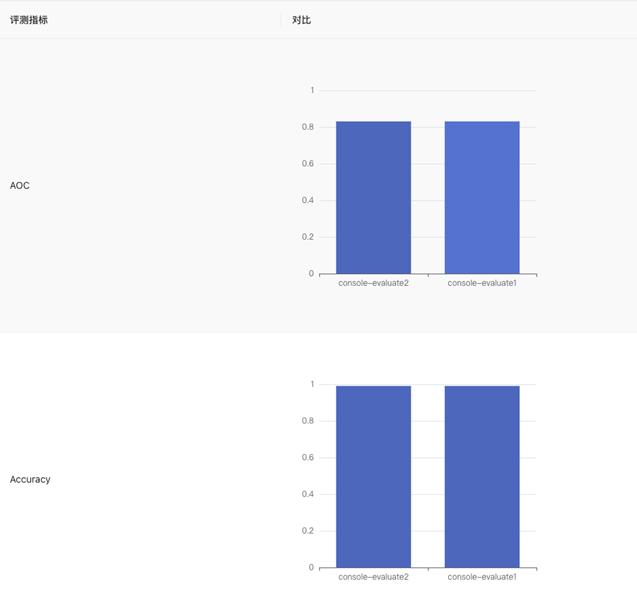This topic describes how to evaluate a model based on metrics such as the accuracy rate and recall rate, and how to view and compare model evaluation results.

Create an evaluation job

1. Access the AI development console
2. In the left-side navigation pane of AI Developer Console, click Model Manage.
3. In the Model Manage List section, find the model that you want to manage and click New Model Evaluate in the Operator column.
4. Set parameters in the EvaluateJob Message and EvaluateJob Config sections.
Section Parameter Description
EvaluateJob Message EvaluateJob Name The name of the evaluation job.
Note The name cannot exceed 256 characters in length, and can contain digits, letters, and hyphens (-). The name is not case-sensitive.
EvaluateJob Image The base image that is used to run the evaluation job.
Namespace The namespace in which the evaluation job runs.
Image Pull Secrets The credentials for pulling private images. This parameter is optional.
Data Configuration The data source configuration. This parameter is optional.

If you want to reference a PVC, you must configure a PVC as the data source.

Model Path The path in which the model that you want to evaluate resides.
Dataset Path The path in which the data set that is used in the evaluation job resides.
Metrics Path The path to which the evaluation metrics are exported.
Execution Command The command that is run in the containers of the evaluation job.
Code Configuration The source code configuration.

If you want to pull the source code for the evaluation job from a Git repository, you must configure a Git repository.

EvaluateJob Config CPU (Cores) The amount of CPU resources used by the evaluation job.
Memory (GB)
GPU (Card Numbers)
The preceding parameters are the same as those when you use Arena to submit an evaluation job. You can use the default evaluation job code or write custom code. For more information about how to write the evaluation job code, see Write evaluation job code.
5. Click Submit Evaluation Job.
On the Evaluate Jobs page, you can view information about the evaluation job that you created.

View evaluation metrics

1. In the left-side navigation pane of the AI development console, click Evaluate Jobs.2. In the Job List section, you can click the name of an evaluation job to view the evaluation metrics.The preceding figure shows the following metrics: Accuracy, Precision, Recall, F1_score, ROC, and AOC. F1_score indicates the average of Precision and Recall. The Receiver Operating Characteristic (ROC) curve shows the performance of the model. The Area Under ROC Curve (AOC) is the basic evaluation metric.

Compare evaluation metrics

1. In the left-side navigation pane of AI Developer Console, click Evaluate Jobs.
2. In the Job List section, select two or more evaluation jobs and click Metrics Compare in the upper-right corner of the section.On the page that appears, the metrics of the selected evaluation jobs are displayed in column charts. This provides a visualized comparison to help you better select from multiple models.Write evaluation job code

Perform the following steps to write custom evaluation job code. For more information about the sample code, see Sample evaluation job code.

1. Run the following command to import the abstract base class (ABC) package and KubeAI package:
from abc
import ABC from kubeai.evaluate.evaluator
import Evaluator from kubeai.api
import KubeAI
2. Define an Evaluator class to inherit the Evaluator abstract class and rewrite the preprocess_dataset, load_model, evaluate_model, and report_metrics methods. These methods are used to preprocess the data set, load the model, evaluate the model, and export the evaluation report.
class CustomerEvaluatorDemo(Evaluator, ABC):
def preprocess_dataset(self): # Preprocess the data set.
def evaluate_model(self, dataset): # Evaluate the model.
def report_metrics(self, metrics): # Export the evaluation model.
3. Create an API client and import the created Evaluator object. Then, call the Evaluate method to run the evaluation job.
customer_evaluator = CustomerEvaluatorDemo()
KubeAI.evaluate(customer_evaluator)
If you want to perform the test on your on-premises machine, you can reference dataset_dir, model_dir, and report_dir to call the Test method to perform an on-premises test.
customer_evaluator = CustomerEvaluatorDemo()
KubeAI.test(customer_evaluator, model_dir, dataset_dir, report_dir)

Sample evaluation job code

The following sample code is used to evaluate the model trained based on the MNIST data set of TensorFlow 1.15.
from kubeai.evaluate.evaluator import Evaluator
from abc import ABC
from kubeai.api import KubeAI
import tensorflow as tf
import numpy as np
from tensorflow.keras import layers, models

class CNN(object):
def __init__(self):
model = models.Sequential()
32, (3, 3), activation='relu', input_shape=(28, 28, 1)))
model.summary()
self.model = model

class TensorflowEvaluatorDemo(Evaluator, ABC):

def preprocess_dataset(self):   # Preprocess the data set.
x_train, y_train = f['x_train'], f['y_train']
x_test, y_test = f['x_test'], f['y_test']

train_images = x_train.reshape((60000, 28, 28, 1))
test_images = x_test.reshape((10000, 28, 28, 1))
train_images, test_images = train_images / 255.0, test_images / 255.0

train_images, train_labels = train_images, y_train
test_images, test_labels = test_images, y_test
"test_images" : test_images,
"test_labels" : test_labels
}

latest = tf.train.latest_checkpoint(self.model_dir)
self.cnn = CNN()
self.model = self.cnn.model

def evaluate_model(self, dataset):  # Evaluate the model.
metrics = Utils.evaluate_function_classification_tensorflow1(model=self.model, evaluate_x=dataset["test_images"], evaluate_y=dataset["test_labels"])
predictions = self.model.predict(dataset["test_images"])
pred = []
for arr in predictions:
pred.append(np.argmax(arr))
pred = np.array(pred)
confusion_matrix = mt.confusion_matrix(dataset["test_labels"], pred)
metrics["Confusion_matrix"] = str(confusion_matrix)
return metrics

def report_metrics(self, metrics):  # Export the evaluation model.
print(metrics)
Utils.ROC_plot(fpr=metrics["ROC"]["fpr"], tpr=metrics["ROC"]["tpr"], report_dir=self.report_dir)
print("Here is the customer-defined report method")

if __name__ == '__main__':
tensorflow_evaluator = TensorflowEvaluatorDemo()  # Create an API client and reference the created Evaluator object. Then, call the Evaluate method to run the evaluation job.
KubeAI.evaluate(tensorflow_evaluator)
# KubeAI.test(tensorflow_evaluator, model_dir, dataset_dir, report_dir)  # Perform the test on your on-premises machine.